# Types of Power

There is a huge amount of false information and misunderstanding regarding types of electrical power, i.e. instantaneous, active, reactive, distortion and apparent. The following article aims to address any misconceptions and provide a solid base for any future analysis on power.

Let’s define what power is!

Instantaneous Power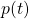, measured in Watts [W], is given by (1) and it is the product of voltage and current, in the time domain.

(1)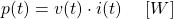This equation is independent of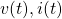waveforms.

Let’s consider that current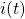, exits from the positive pole of the source. Positive value ofmeans that power flow is from the power source to the load, while negative means the opposite, as can be seen in the figure below.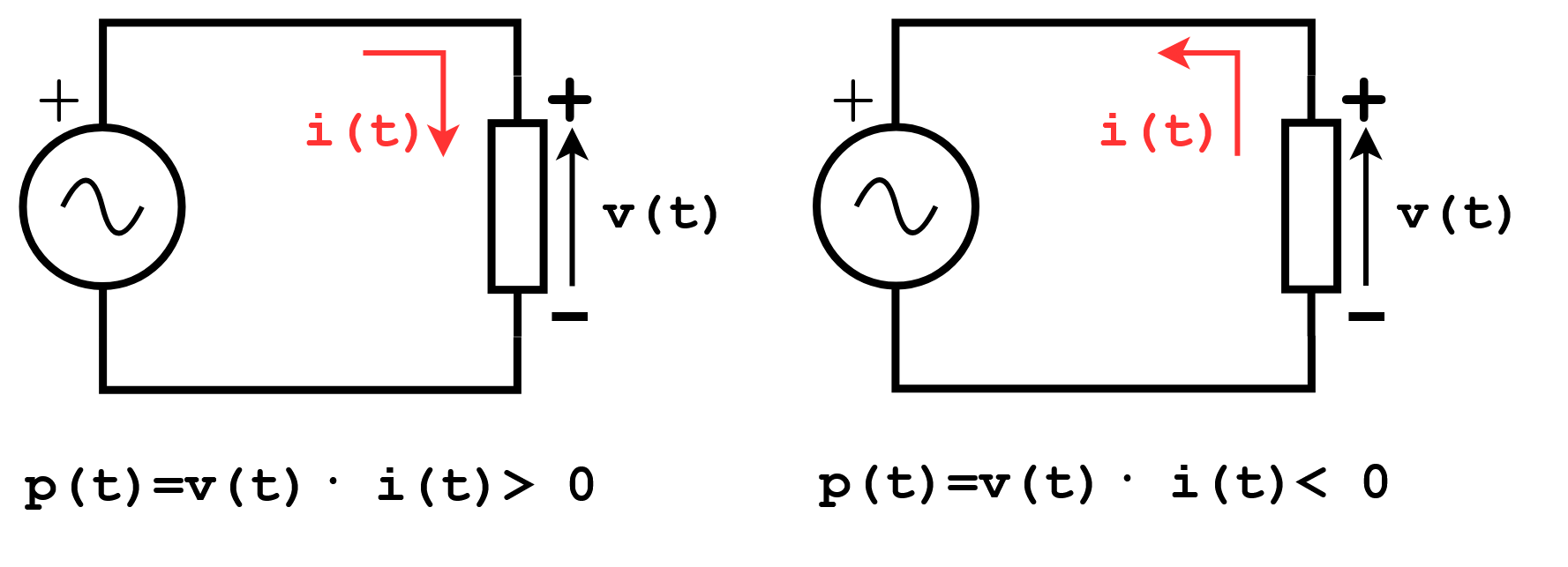But how is possible for a load to transfer power to a source?

There are some mechanism for this:

• Energy stored in electric or magnetic fields, generated by the load, i.e. capacitors and inductors
• Power transformed to electrical from another form, i.e. an electrical machine that rotates
• In this category we can include batteries, with store chemical energy and with some proper peripherals can either be charged or supply power
• Power Electronic Converters that use non-linear components, such as diodes, thyristors and transistors and distort the signals, resulting to power oscillations

So, how do we know how much energy or power was transferred from the source to the load, during a time interval? We integrate!

Active Power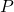, is the integral of instantaneous power, during some time interval, as defined in equation (2)

(2)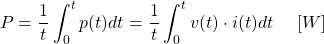Now, ifis a periodic signal, if for example it is the product of two sinusoidal voltage and current waves, then we define, as shown in equation (3)

(3)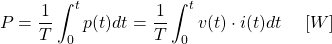Does this makes sense from dimensions perspective? Well, sure.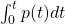is measured in Joules [J] and divided by time [s],is measured in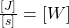.